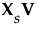Launch the Principal Components platform by selecting Analyze > Multivariate Methods > Principal Components. Principal Component analysis is also available using the Multivariate and the Scatterplot 3D platforms.
The example described in Example of Principal Component Analysis uses all of the continuous variables from the Solubility.jmp sample data table.
Principal Components Launch Window
 •
 •
The Default option uses either the Row-wise, Pairwise, or REML methods:
 • Row-wise is used for data tables with no missing values.
 • Pairwise is used in the following circumstances:
 ‒ the data table has more than 10 columns or more than 5,000 rows and has missing values
 ‒ the data table has more columns than rows and has missing values
 • REML is used otherwise.
 • Checking compatibility with JMP versions earlier than JMP 8. Row-wise estimation was the only estimation method available before JMP 8.
 • Excluding any observations that have missing data.
 • n = number of rows
 • p = number of variables
 • X = n by p matrix of data values
When you select the Wide method, the data are standardized. To standardize a value, subtract its mean and divide by its standard deviation. Denote the n by p matrix of standardized data values by Xs. Then the covariance matrix of the standardized data is the correlation matrix of X and it is given as follows:
Using the singular value decomposition, Xs is written as UDiag(Λ)V’. This representation is used to obtain the eigenvectors and eigenvalues of Xs’Xs. The principal components, or scores, are given by.